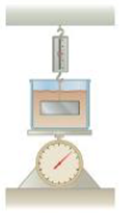Chapter 9, Problem 31P

Chapter
Section
Textbook Problem

A 1.00-kg beaker containing 2.00 kg of oil (density = 916 kg/m3) rests on a scale. A 2.00-kg block of iron is suspended from a spring scale and is completely submerged in the oil (Fig. P9.31). Find the equilibrium readings of both scales.Figure P9.31

To determine
The equilibrium readings of both scales.

Explanation

Section1:

To determine: The equilibrium reading of the upper scale.

Answer: The equilibrium reading of the upper scale is 17.3N .

Explanation: The volume of the iron block is V=miron/ρiron and the buoyancy force on the block is B=ρoilVg=ρoil(miron/ρiron)g . Using Newton’s equations of motion at equilibrium condition, the upper scale reading would be Fy=0=Fupper+BmirongFupper=miron[1(ρoil/ρiron)]g .

Given info: The mass of iron block is 2.00kg , acceleration due to gravity is 9.80m/s2 , density of iron is 7.86×103kg/m3 , and the density of oil is 916kg/m3 .

The formula for the upper scale reading is,

Fupper=miron[1ρoilρiron]g

• miron is mass of iron.
• g is acceleration due to gravity.
• ρiron is density of iron.
• ρoil is density of oil.

Substitute 2.00kg for miron , 9.80m/s2 for g , density of oil is 7.86×103kg/m3 , 7.86×103kg/m3 for ρiron , and 916kg/m3 for ρiron to find Fupper .

Fupper=(2.00kg)(1916kg/m37.86×103kg/m3)(9.80m/s2)=17.3N

Thus, the equilibrium reading of the upper scale is 17.3N .

Section2:

To determine: The equilibrium reading of the lower scale.

Answer: The equilibrium reading of the lower scale is 31.7N .

Explanation: Use Newton’s equation of motion as FlowerB(moil+mbeaker)g=0 and it is rearranged for the lower scale reading is Flower=[ρoil(miron/ρiron)+(moil+mbeaker)]g

Still sussing out bartleby?

Check out a sample textbook solution.

See a sample solution

The Solution to Your Study Problems

Bartleby provides explanations to thousands of textbook problems written by our experts, many with advanced degrees!

Get Started